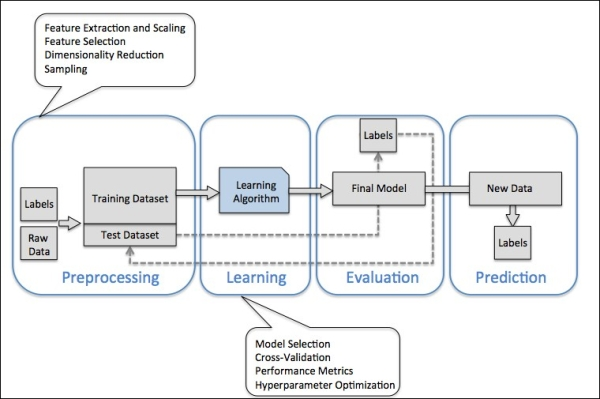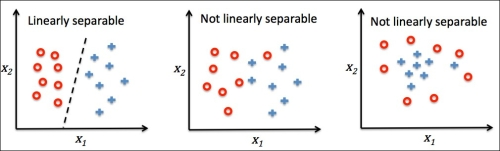Topics: AI | Data Science | Data Visualization | Deep Learning | Machine Learning | NLP | Python | R | Statistics

KDnuggets Home » News » 2017 » Feb » Tutorials, Overviews » What I Learned Implementing a Classifier from Scratch in Python

# What I Learned Implementing a Classifier from Scratch in Python

http likes 398

In this post, the author implements a machine learning algorithm from scratch, without the use of a library such as scikit-learn, and instead writes all of the code in order to have a working binary classifier algorithm.

By Jean-Nicholas Hould, JeanNicholasHould.com.

This post is part of the author's Learning Machine Learning series. It’s based on Chapter 1 and 2 of Python Machine Learning.

Machine learning can be intimidating for a newcomer. The concept of a machine learning things alone is quite abstract. How does that work in practice?

In order to demystify some of the magic behind machine learning algorithms, I decided to implement a simple machine learning algorithm from scratch. I will not be using a library such as scikit-learn which already has many algorithms implemented. Instead, I’ll be writing all of the code in order to have a working binary classifier algorithm. The goal of this exercise is to understand its inner workings.

### So, what the heck is a binary classifier?

A classifier is a machine-learning algorithm that determines the class of an input element based on a set of features. For example, a classifier could be used to predict the category of a beer based on its characteristics, it’s “features”. These features could include its alcohol content, aroma, appearance, etc. A machine learning classifier could potentially be used to predict that a beer with 8% alcohol content, 100 IBU and with strong aromas of oranges is an Indian Pale Ale.

In machine learning, there are three main types of tasks: unsupervised learning, supervised learning and reinforcement learning. The classifier algorithm falls under the supervised learning category. Supervised learning means that we know the right answer beforehand. The desired outputs are known. In the case of the beer example, we could realistically have a dataset describing beers and their category. We could train the classifier algorithm to predict those categories based on the beers features.

A binary classifier classifies elements in two groups. Zero or one. True or false. IPA or not.

### Building a machine learning model

There are four steps to build and use a machine learning model.

1. Preprocessing
2. Learning
3. Evaluation
4. PredictionSource: Python Machine Learning by Sebastian Raschka.

### Preprocessing

The preprocessing is the first step in building a machine learning model. At this step, you acquire and prepare the data for future usage. You clean up the data, tidy it and select the features you want to use from your data.

The following tasks can be considered as part of the “preprocessing”:

• Extract features from raw data
• Clean and format the data
• Remove superfluous features (or highly correlated features)
• Reduce the number of features for performance
• Standardize the range of feature data (also named Feature Scaling)
• Split your dataset randomly: training dataset and test dataset

### Learning or Training

Once you have your datasets ready to be used, the second step is to select an algorithm to perform your desired task. In our case, the algorithm we selected is a binary classifier called Perceptron. There are many algorithms designed to do different tasks. They each have their strengths and weaknesses.

At this step, you can test a few algorithms, see how they perform and select the best performing one. There are a wide variety of metrics that can be used to measure the performance of a machine learning model. According to Raschka, “one commonly used metric is classification accuracy, which is defined as the proportion of correctly classified instances”. At this step, you will make adjustments to the parameters of your machine learning algorithm. These are named hyperparameters.

In this post, we’ll mainly focus on this part of the machine learning work flow. We’ll deep dive in the algorithm inner workings. If you are interested in the other sections of the machine learning work flow, which you should be, I’ll be linking to a great notebook at the end of this post.

### Evaluation

When the model has been “trained” on the dataset it can be evaluated on new unseen data. The goal here is to measure the generalization error. This metric measures “how accurately an algorithm is able to predict outcome values for previously unseen data”. Once you are satisfied with the results, you can use your machine learning model to make predictions.

### Introducing the Perceptron

The algorithm that we’ll be re-implementing is a Perceptron which is one of the very first machine learning algorithm.

The Perceptron algorithm is simple but powerful. Given a training dataset, the algorithm automatically learns “the optimal weight coefficients that are then multiplied with the input features in order to make the decision of whether a neuron fires or not”.

But, how does the algorithm do that?

### The Algorithm

Here’s the sequence of the algorithm:

First, we initialize an array with the weights equal to zero. The array length is equal to the number of features plus one. This additional feature is the “threshold”. It’s important to note that in the case of the Perceptron algorithm, the features must be of numerical value.

``````
self.w_ = np.zeros(1 + X.shape)
``````

Secondly, we start a loop equal to the number of iterations `n_iter`. This is an hyperparameter defined by the data scientist.

``````
for _ in range(self.n_iter):
``````

Thirdly, we start a loop on each training data point and it’s target. The target is the desired output we want the algorithm to eventually predict. Since this is a binary classifier, the targets are either `-1` or `1`. They are of binary value.

Based on the data point features, the algorithm will predict the category: `1` or `-1`. The prediction calculation is a matricial multiplication of the features with their appropriate weights. To this multiplication we add the value of the threshold. If the result is above 0, the predicted category is `1`. If the result is below 0, the predicted category is `-1`.

At each iteration on a data point, if the prediction is not accurate, the algorithm will adjust the weights. During the first few iterations, the predictions are not likely to be accurate because the weights haven’t been adjusted many times. They haven’t had a chance to start converging. The adjustments are made proportionally to the difference between the target and the predicted value. This difference is then multiplied by the learning rate `eta`, an hyperparameter of value between zero and one set by the data scientist. The higher the `eta` is, the larger the correction on the weights will be. If the prediction is accurate, the algorithm won’t adjust the weights.

The Perceptron will converge only if the two classes are linearly separable. Simply said, if you are able to draw a straight line to entirely separate the two classes, the algorithm will converge. Else, the algorithm will keep iterating and will readjust weights until it reaches the maximum number of iterations `n_iter`.Source: Python Machine Learning by Sebastian Raschka.

### Complete Code

Source: Python Machine Learning by Sebastian Raschka.

### 1. Learning Rate, Number of Iteration & Convergence

Parameters such as `learning rate` and `number of iteration` can seem very abstract if you jump in straight to using an algorithm from a library like `scikit-learn`. It’s hard to grasp what these really do. By implementing the algorithm, it’s now clear for me what they represent in the context of the Perceptron.

Learning Rate

The learning rate is a ratio by which the weights are corrected when the prediction is not accurate. The value needs to be between zero and one. As you can see in the snippet below, the `fit` function will iterate on each observation, call the `predict` function and then adjust the weights based on the difference between the target and the predicted value and then multiplied by the learning rate.

A higher learning rate means that the algorithm will adjust the weights more aggressively. At each iteration, the weights will be adjusted if the predicted value is inaccurate.

Number of iterations

The number of iteration is the number of times the algorithm will run through the training dataset. If the number of iteration was set to one, the algorithm would loop through the dataset only once and update the weights one time for each data point. The resulting model would be more likely to be inaccurate than a model with a higher number of iteration. On large datasets, there is a cost to having a high number of iterations.

The `learning rate` and `number of iteration` go hands-in-hand. They need to be adjusted together. For example, if you have a very low `learning rate`, which means that the algorithm will adjust it’s weight only marginally at each iteration, you will probably need a higher number of iteration.

### 2. Linear Algebra

It’s critical to mention that the capabilities of the Perceptron algorithm are attributable to linear algebra. The whole algorithm can be described through linear algebra formulas. If you have never done linear algebra in college, the formulas will be cryptic. As usual, Khan Academy is a great place to start with if you want to get familiar with linear algebra. It’s also a great place to get a refresher on the topic.

For me, the main learning here is how fundamental linear algebra is to this machine learning algorithm.

### 3. Type Everything

This learning is actually a concept I re-learned while going through the code for this post. It’s not specific to machine learning and it has nothing to do with the Perceptron.

Back in 2012 when I was learning to code Ruby on Rails, a web application development framework, I realized that typing down all of the code examples from tutorials really helped me memorize and understand the concepts. I spent weeks writing code while following tutorials. No copy and paste. I typed all the code. This may sound stupid but it was extremely helpful to grasp the concepts. During the process, I inevitably made typos and spend some time figuring out what was broken. These moments were crucial because that’s when you usually stop and think.

If you are going through the Perceptron code, don’t copy and paste the code from the repository. Type it down in your own Jupyter Notebook. Type everything. Don’t read passively. Get involved, type it down and you’ll assimilate the concepts faster.

### Next Steps

In this post, my goal was to share my understanding of the algorithm and the learnings I’ve made while reimplementing it. However, you can do much more than simply reimplementing the model. You can actually use it with real data in order to do some simple predictions. In Python Machine Learning, Raschka uses the Perceptron to predict the class of Iris flower based on a the sepal and petal length of the flower. With actual data, you can then evaluate the model and make predictions on unseen data.

Bio: Jean-Nicholas Hould is a Data Scientist from Montreal, Canada. Author at JeanNicholasHould.com.

Original. Reposted with permission.

Related:Get KDnuggets, a leading newsletter on AI, Data Science, and Machine Learning